Solving Linear Inequalities:
Introduction and Formatting
(page 1 of 3)

Sections: Introduction and formatting, Elementary examples, Advanced examplesSolving linear inequalities is almost exactly like solving linear equations.

• Solve x + 3 < 0.

If they'd given me "x + 3 = 0", I'd have known how to solve: I would have subtracted 3 from both sides. I can do the same thing here: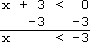Then the solution is:

x < −3

The formatting of the above answer is called "inequality notation", because the solution is written as an inequality. This is probably the simplest of the solution notations, but there are three others with which you might need to be familiar.   Copyright © Elizabeth Stapel 2002-2011 All Rights Reserved

"Set notation" writes the solution as a set of points. The above solution would be written in set notation as "{x | xis a real number, x
< −3}
", which is pronounced as "the set of all x-values, such that x is a real number, and x is less than minus three". The simpler form of this notation would be something like "{x | x
< −3}
", which is pronounced as "all x such that x is less than minus three".

"Interval notation" writes the solution as an interval (that is, as a section or length along the number line). The above solution, "x < −3", would be written as "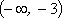", which is pronounced as "the interval from negative infinity to minus three", or just "minus infinity to minus three". Interval notation is easier to write than to pronounce, because of the ambiguity regarding whether or not the endpoints are included in the interval. (To denote, for instance, "x < −3", the interval would be written "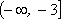", which would be pronounced as "minus infinity through (not just "to") minus three" or "minus infinity to minus three, inclusive", meaning that −3 would be included. The right-parenthesis in the "x < −3" case indicated that the −3 was not included; the right-bracket in the "x < −3" case indicates that it is.)

The last "notation" is more of an illustration. You may be directed to "graph" the solution. This means that you would draw the number line, and then highlight the portion that is included in the solution. First, you would mark off the edge of the solution interval, in this case being −3. Since −3 is not included in the solution (this is a "less than", remember, not a "less than or equal to"), you would mark this point with an open dot or with an open parenthesis pointing in the direction of the rest of the solution interval: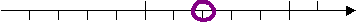...or: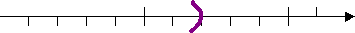Then you would shade in the appropriate side: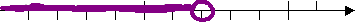...or: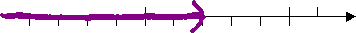Why shade to the left? Because they want all the values that are less than−3, and those values are to the left of the boundary point. If they had wanted the "greater than" points, you would have shaded to the right.

In all, we have seen four ways, with a couple variants, to denote the solution to the above inequality:

 notation format pronunciation inequality x < −3 x is less than minus three set i) {x | x is a real number, x < −3} ...or: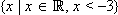ii) {x | x < −3} i) the set of all x, such that x is a real number and xis less than minus three ii) all x such that x is less than minus three intervalthe interval from minus infinity to minus three graph either of the following graphs:Here is another example, along with the different answer formats:

• Solvex − 4 > 0.

If they'd given me "x − 4 = 0", then I would have solved by adding four to each side. I can do the same here: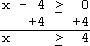Then the solution is: x > 4

Just as before, this solution can be presented in any of the four following ways:

 notation format pronunciation inequality x > 4 x is greater than or equal to four set i) {x | x is a real number, x > 4} ...or: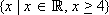ii) {x | x> 4} i) the set of all x, such that x is a real number, and x is greater than or equal to four ii) all x such that x is greater than or equal to four interval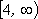the interval from four to infinity, inclusive of four graph either of the following graphs: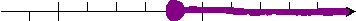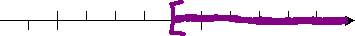Regarding the graphs of the solution, the square bracket notation goes with the parenthesis notation, and the closed (filled in) dot notation goes with the open dot notation. While your present textbook may require that you know only one or two of the above formats for your answers, this topic of inequalities tends to arise in other contexts in other books for other courses. Since you may need later to be able to understand the other formats, make sure now that you know them all. However, for the rest of this lesson, I'll use only the "inequality" notation; I like it best.

Top  |  1 | 2 | 3  |  Return to Index  Next >>

 Cite this article as: Stapel, Elizabeth. "Solving Linear Inequalities: Introduction and Formatting." Purplemath. Available from     https://www.purplemath.com/modules/ineqlin.htm. Accessed [Date] [Month] 2016

MathHelp.com Courses